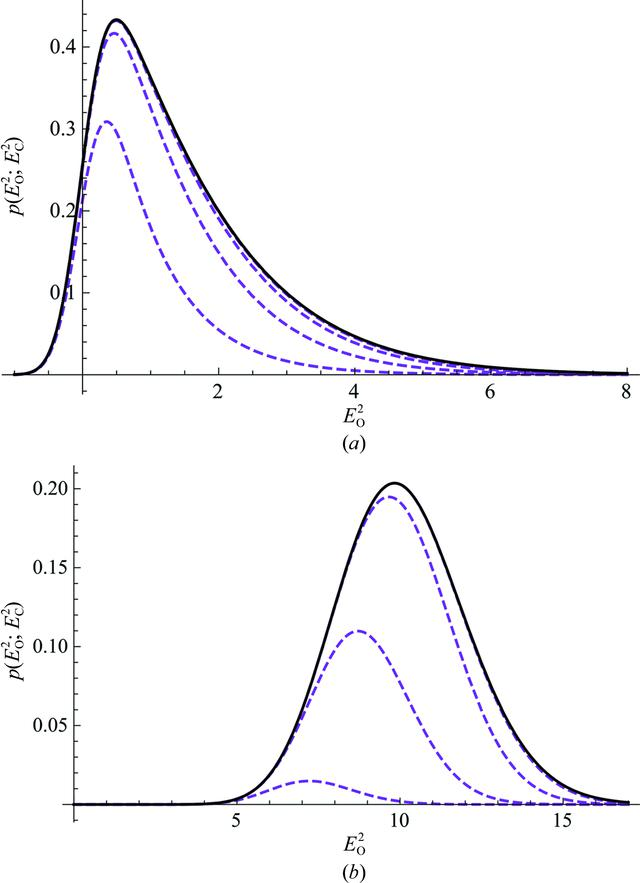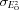disable zoom     view article Figure 2 Illustration of the convergence of the MLI approximation (Pannu & Read, 1996) to the exact intensity likelihood function for the acentric case (6b). Approximations with increasing numbers of terms are shown as dashed magenta lines, while the exact function is shown in black. In both examples, the approximation with the largest number of terms is almost indistinguishable from the exact function. (a) Example with EC = 2.0, σA = 0.4 and= 0.3. The dashed magenta curves show approximations with terms to order 0, 1, 2 and 3. (b) Example with EC = 3.5, σA = 0.9 and= 0.3. The dashed magenta curves show approximations with terms to order 40, 50, 60 and 70.STRUCTURALBIOLOGY
ISSN: 2059-7983
Volume 72| Part 3| March 2016| Pages 375-387